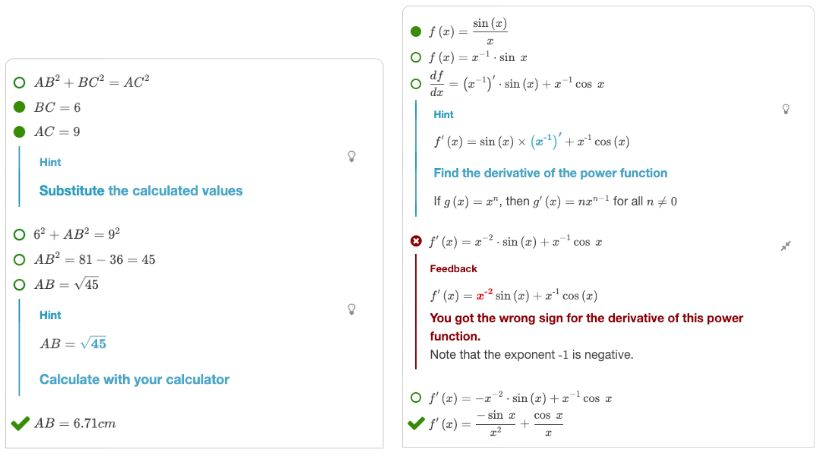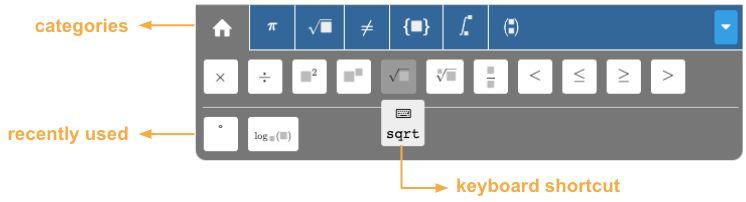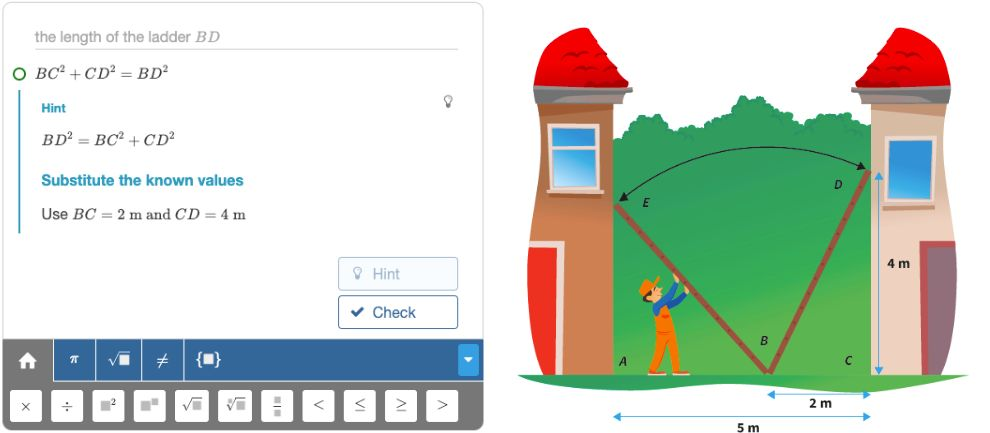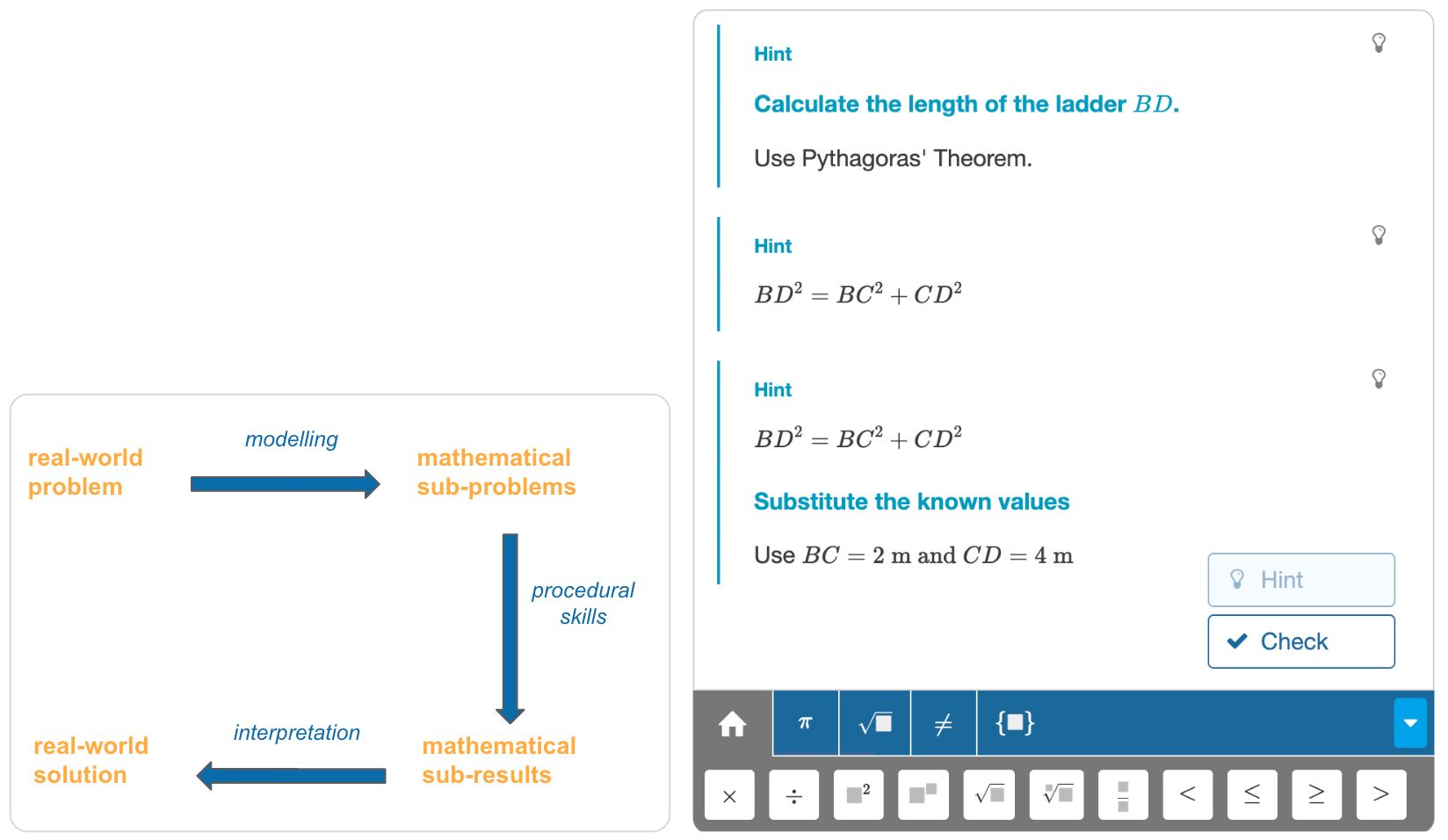# Multistep

## What is the Multistep question type?

The Multistep question type applies to questions that require mathematical expressions to derive the result. It is especially well suited to problems that require a multistep approach, hence its name.

You can use Multistep for many different subjects in math. The figure below shows two examples: A question about applying the Pythagoras theorem and a question about differentiation.Figure: Using Multistep on a Pythagoras problem and a calculus problem.

Note how Algebrakit evaluates all steps, not just the final answer. Multistep can generate personalized hints and error feedback as well. "Personalized" means that the hints and error feedback are not just general directives but apply to the student's work.

The question below uses Multistep to offer a question about a linear equation. It is a live question, so go ahead and try if you can solve this one!

## Features

### Formula editor

In a Multistep question, the student often has to input multiple mathematical expressions, and we want this to be quick and easy. That's why the formula editor offers some smart features to facilitate input:Figure: Buttons of the formula editor.

• There are buttons for all relevant operations organized in categories.
• The home category contains the most-used buttons and automatically adds any symbols you have recently used.
• You can type most operations and even complex expressions with the keyboard.
• You can quickly copy and change previous inputs using the arrow keys. You can also copy and paste using the mouse.

### Multi-part and story problems

You can go beyond mathematical derivations and use Multistep for more elaborate exercises, such as story problems. The solutions to such problems often include multiple and interdependent derivations.Figure: A fragment from a story problem. "At what height does the ladder touch the left wall?"

The solution process of a complex problem goes through several phases of thinking. During modeling, you decompose a complex problem into smaller parts. Mathematization is about transforming each part into a mathematical formulation, such as a formula or an equation. Next, procedural skills are needed to solve these mathematical problems. The results are finally combined and interpreted to get the final solution.Figure: Left: The solution process of story problems. Right: Three multi-layer hints.

### Multi-layer hints

The Multistep question type has a sophisticated, multi-layer hint system that follows this solution process.

1. Modeling: Indicating what to calculate next in natural language.
• E.g., "Calculate the length of the ladder."
2. Mathematization: Showing the corresponding mathematical expression.
• E.g., $BC^2+CD^2=BD^2$
3. Procedural: Describing the next mathematical step.
• E.g., "Substitute the known values."

Each subsequent hint goes down to the next level of detail. Algebrakit will not give away the result of a step, so it is not possible to retrieve answers by just clicking the hint button repeatedly.

### Assessment mode

Of course, students sometimes have to prove they can solve math problems without support. That's why the Algebrakit question types can run in an assessment mode. For Multistep, this means that sub-step evaluation, hints, and error feedback are disabled, although the student can still write multistep derivations. The sub-step evaluation and error feedback are available after submitting the question or test.

## Applicable math topics

Multistep applies to a very broad range of math problems. Examples are:

• Arithmetic: order of operations, negative numbers, reducing or rewriting fractions, fraction arithmetic, etc.
• Algebra: expanding parentheses, factoring, simplifying complex expressions, rewriting fractions, power functions, roots, trigonometric functions, etc.
• Equations: linear, quadratic, hyperbolic, trigonometric, exponential, and other types of equations. Inequalities and systems of two equations. Systems of more than two equations are currently not supported.
• Calculus: Finding the derivative, anti-derivative, integral, or limit. Using the chain, quotient, and product rules for differentiation.

Multistep is about solving a problem and is less suited to evaluate mathematical proof. For example, Multistep cannot handle a math problem like "Proof that $\sqrt{2}$ is a real number."